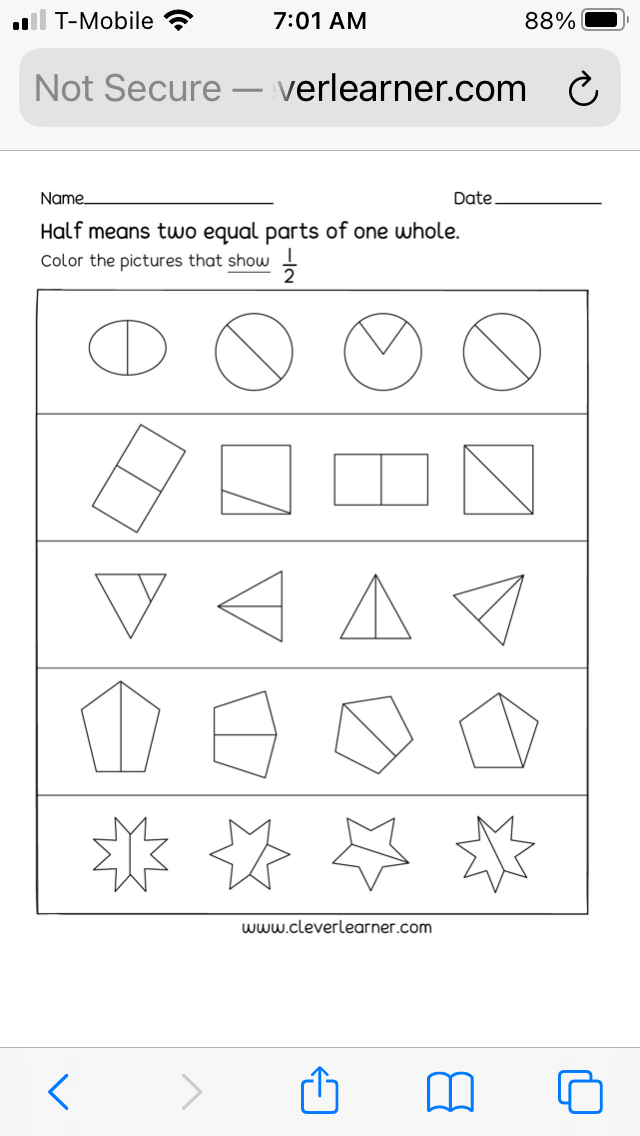Author:
Crescent
Subject:
Elementary Mathematics
Material Type:
Lesson Plan
Level:
Lower Elementary
Tags:
Lesson Plan
Language:
English
Media Formats:
Text/HTML

# Creating fractions 1/2 and 1/4## Overview

This lesson plan will cover in part standard 2.G.3. We will introduce the terms "half" and "fourths". Students will discriminate between equally divided wholes and nonequally divided wholes. Students will be assessed to determine if they can accurately identify and divide into halves and fourths.

# Summary:

Students will identify wholes divided equally and nonequally.

Students will divide in halves and fourths.

45 minutes lesson plan and assessment.

Face-to face instruction.

# Background for Teachers

To teach this lesson, you will need to be able to accurately divide wholes into halves and fourths using a whiteboard or smartboard features. You will also identify students who will need diverse activities and assessments.

You will need to be familiar with Lucid for Education to guide students through a collaborative activity.

# Step 1 - Goals and Outcomes

Learning Intentions:

• Students will be able to accurately identify wholes divided into equal parts versus wholes divided unequally.
• Students will be able to divide wholes into halves.
• Students will be able to further divide halves into fourths.
• Students will use the terms halves and fourths to correctly identify parts of a whole.

# Step 2 - Planning Instruction

Prior to this lesson, students will need to have an understanding of equal meaning the same amount. Use the assessment under the assessment section by clever learner.

Strategies for Diverse Learners

Students can show understanding by vocally identifying halves and fourths from wholes someone else divides pr color in 1/4 and 1/2 of divided objects.

Students can use scissors to divide or draw a line to divide.

Teacher-

Gather 4-5 pictures of objects that can easily be divided equally and 2-3 pictures of items unable to be divided equally.

Access Lucid collaborative sheet for students to work in pairs

Print assessment for individuals.

# Step 3 - Instruction

Begin with the scenario of 2 students are given one large cookie. How can they divide it to share equally? Use pictures you collected to show wholes divided equally and nonequally. Ask students to identify which wholes are divided equally.

Introduce the term "half" to mean 2 equal parts of a whole. Practice naming which pictures above are divided "in half".

Ask for students help to divide more whole objects in half gradually working to the more abstract items of shapes drawn on whiteboard. Use dividing shapes in half equally.

Pair students up to complete lucid collaborative sheet in halves and correct before moving on to fourths. Fourths can be made by using the collaborative lucid sheet students created 1/2 on. Fourths are halves divided again.

# Step 4 - Assessments

Students will be assessed in several ways.

Students will be given a prior assessment attached below to determine equal parts.

Partner work on Lucid sheet dividing in halves and fourths will be recorded.

Students will be assessed through observation if they require vocal assessment rather than written.

Students will be assessed through printed assessment of dividing shapes in halves and fourths independently.

Students can use precut shapes to identify which are cut into halves, fourths and which are not cut equally.

cleverlearner.com and freeprintableonline.com provide the attached worksheets for free to use in your classroom. Only print for immediate use by your children, classroom students or for children in any setting with no commercial intent.